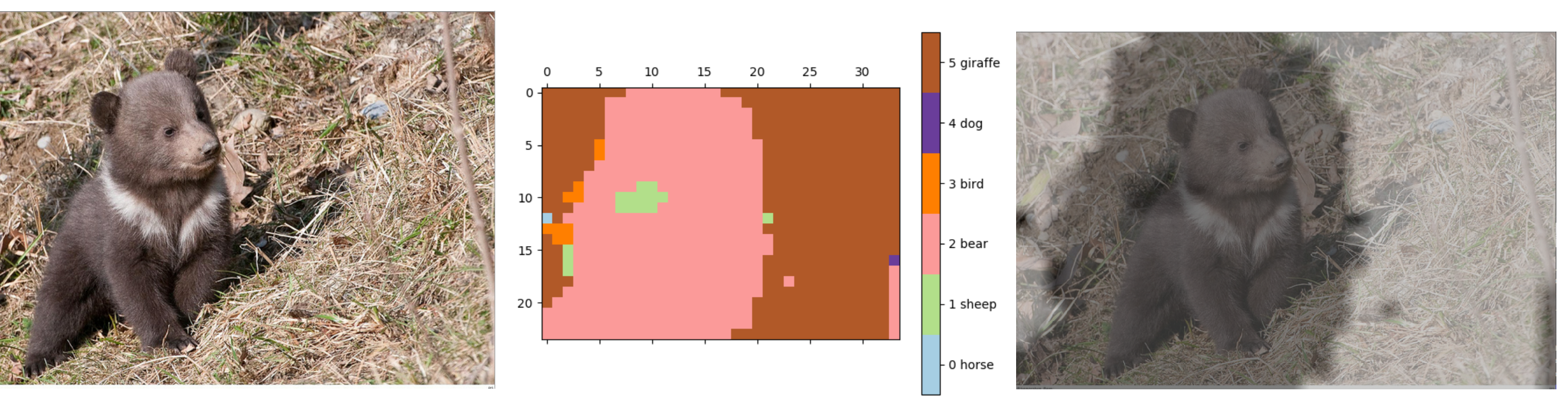# Fully convolutional networks for semantic segmentation

## Overview

A post showing how to perform Image Classification and Image Segmentation with a TF-Slim library and pretrained models. For the simplicity, we would do segmentation using an existing Convolutional Neural Network by applying it in a Fully Convolutional manner, this way we can input image of any size and get segmentation of lower resolution due to max-pooling layers that are used in network. To get the Segmentation of the same size as an input, deconvolutional layers can be used. You can read more about this in the paper fully convolutional networks for semantic segmentation by Long et al. We won’t consider deconvolutional layers in this example, if you would like to look at a FCNN using deconvolutional layers take a look at my Road Semantic Segmentation Project.

## Inroduction

TF-slim is a high level library to help make easy implementation of standard pretrained models like ResNet, VGG, Inception-ResNet-v2 and others. Slim is a very clean and lightweight wrapper around Tensorflow with pretrained models.

Download the VGG-16 model which we will use for classification of images and segmentation. You can also use networks found in TF-slim nets.

Lets take a pick at TF-slim vgg16 source code. As you can see in the code bellow (line 205-217) you can see all of this if statements like ‘if global_pool’ and others. Those are options calls when we initialize our model. In our case is important to look at spatial_squeeze option because this option would allow us to use this net as fully conected layers.

      if global_pool:
net = tf.reduce_mean(net, [1, 2], keep_dims=True, name='global_pool')
end_points['global_pool'] = net
if num_classes:
net = slim.dropout(net, dropout_keep_prob, is_training=is_training,
scope='dropout7')
net = slim.conv2d(net, num_classes, [1, 1],
activation_fn=None,
normalizer_fn=None,
scope='fc8')
if spatial_squeeze:
net = tf.squeeze(net, [1, 2], name='fc8/squeezed')
end_points[sc.name + '/fc8'] = net


## Implementation

#### Load vgg16 using tensorflow slim

    with slim.arg_scope(vgg.vgg_arg_scope()):
# spatial_squeeze option enables to use network in a fully convolutional manner
logits, _ = vgg.vgg_16(input_image,
num_classes=len(labels_name),
is_training=False,
spatial_squeeze=False)


Because we are skipping the fully conected layers and softmax our output would be a vector of class prediction for each pixel. To be more precise, these are not probabilities, because we didn’t apply softmax. But if we pick a class with the highest value it will be equivalent to picking the highest value after applying softmax. If we would like to classified the image we would need to pick the one with the highest probability, we can do this with argmax to returns the index with the largest value across axes of a tensor.

    pred = tf.argmax(logits, dimension=3)

# init_fn = slim.assign_from_checkpoint_fn(model_path, slim.get_model_variables('vgg_16'))
variables_to_restore = tf.contrib.framework.get_variables_to_restore()
init_fn = tf.contrib.framework.assign_from_checkpoint_fn(model_path, variables_to_restore)

new_segmentation = tf.expand_dims(pred, -1)
image_segmentation = tf.image.resize_images(new_segmentation, [tf.shape(image), tf.shape(image)])
image_segmentation = tf.squeeze(image_segmentation, [0, -1])

with tf.Session() as sess:
init_fn(sess)  # load the pre-trained weights
segmentation, np_image, np_logits, image_segmentation = sess.run([pred, image, logits, image_segmentation])



## Output image:## Reference